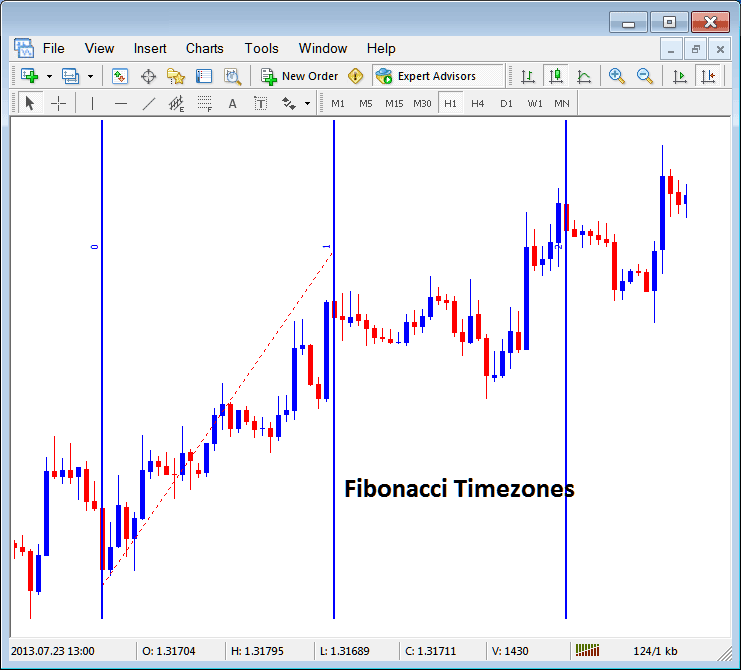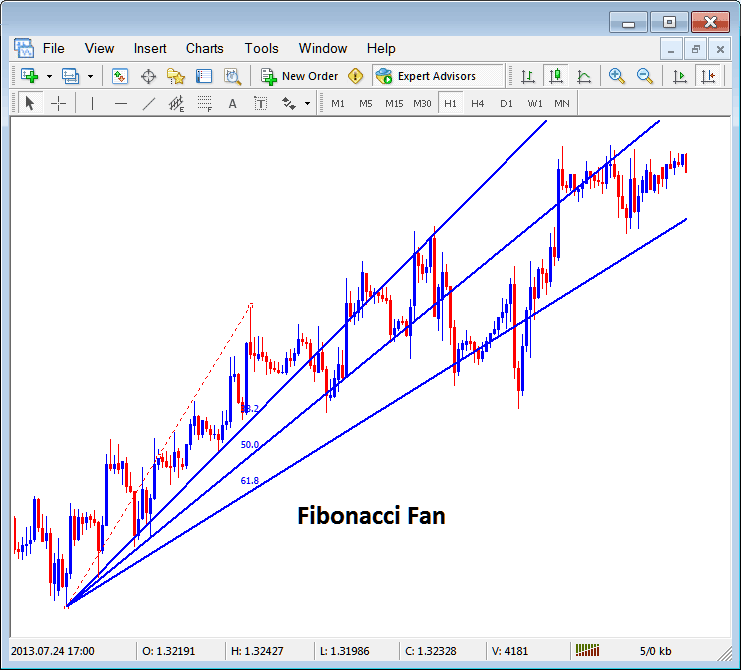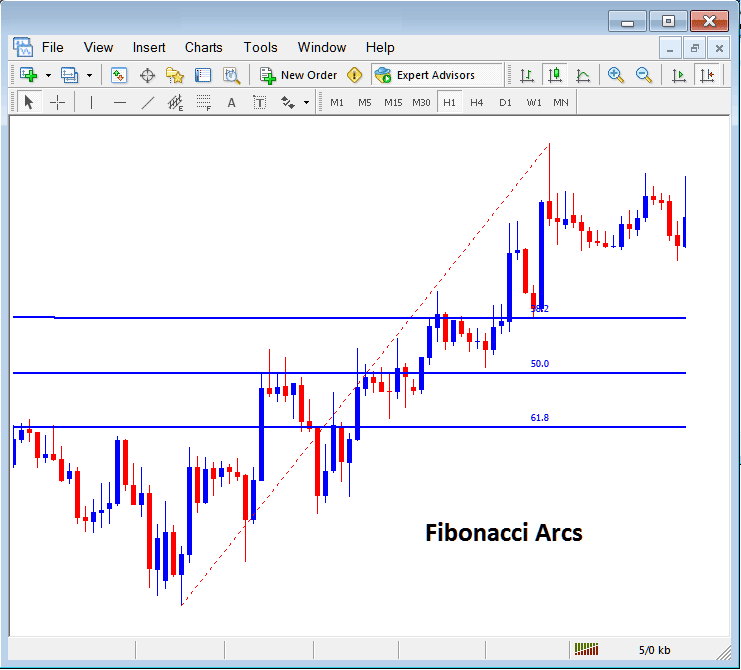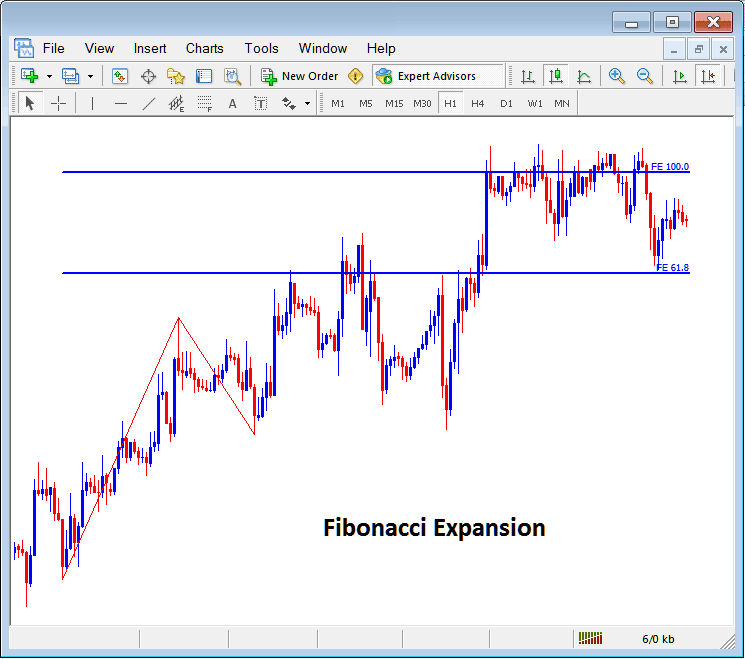Learn Gold Trading for Beginners Tutorials

# Placing Fibonacci Lines On MetaTrader 4 XAUUSD Platform

The fifth option on the MetaTrader 4 gold trading platform insert menu is Fibonacci Lines. The Fibonacci Lines Button has the following sub menu buttons shown below:## Fibonacci Lines

1. Gold Fibonacci Retracement
2. Fibonacci Time Zones
3. Fibonacci Fan
4. Fibonacci Arcs
5. XAUUSD Fibonacci Expansion

## Gold Fibonacci Retracement

The Fibonacci retracement can be placed on the a XAUUSD Trading chart from the Fibonacci retracement button. Fibonacci retracement is used to estimate where a xauusd price retracement is likely to reach. The Fibonacci retracement levels are drawn as shown below. To learn how to draw and use Gold Fibonacci Retracement Levels read the tutorial: How to draw Gold Fibonacci Retracement Levels:Gold Fibonacci Retracement Levels Drawn on a Gold Chart on The MetaTrader 4 XAUUSD Platform

## Fibonacci Time Zones

The Fibonacci time zones are Fibonacci lines drawn at different Fibonacci timelines, these Fibonacci time zones are calculated using the Fibonacci points 1, 2, 3, 5, 8, 13, 21, 34. The Fibonacci levels are 1+2=3, 2+3=5, 3+5=8, first number add to the next, the next added to the second and so on.

The Fibonacci time zones are shown below, at these time lines gold price changes are likely to occur based on technical analysis of Fibonacci time zones:## Fibonacci Fan

The Fibonacci fan is drawn between two chart points and then three additional lines below the xauusd trend are drawn as the fans, these are 38.2%, 50.0% and 61.8% Fibonacci retracement levels. The gold price is likely to bounce and continue in the original direction after gold price retraces to any of these fan lines.

The Fibonacci Fan Lines are drawn as shown below:## Fibonacci Arcs

Fibonacci arcs are drawn using two chart points, the arcs are then drawn as shown below and these arcs represent 38.2%, 50.0% and 61.8% Fibonacci retracement levels.Fibonacci Arcs Representing Gold Fibonacci Retracement Zones on a XAUUSD Chart

## XAUUSD Fibonacci Expansion

The Fibonacci expansion is used to determine the most probable level at which traders should set their take profit levels based on Fibonacci expansion level 61.8% and 100.0% expansion levels. The Fibonacci expansion levels are also referred to as XAUUSD Fibonacci Extension Levels or Fibonacci Projection Levels by some traders and these XAUUSD Fibonacci Extension levels are drawn as shown below. Tutorial: How to Draw XAUUSD Fibonacci Expansion Levels:How to draw Fibonacci Lines and other Fibonacci studies like XAUUSD Fibonacci Expansion and Retracement on MT4 XAUUSD Trading Platform